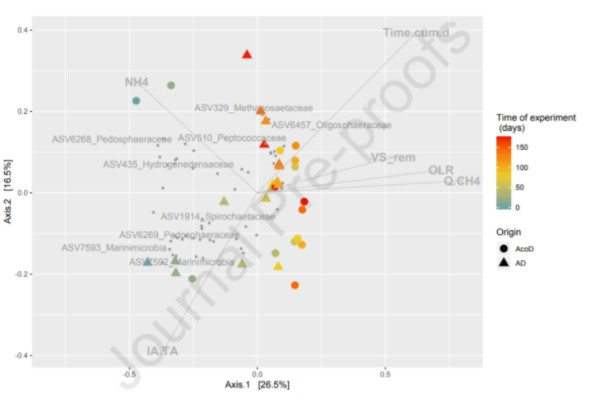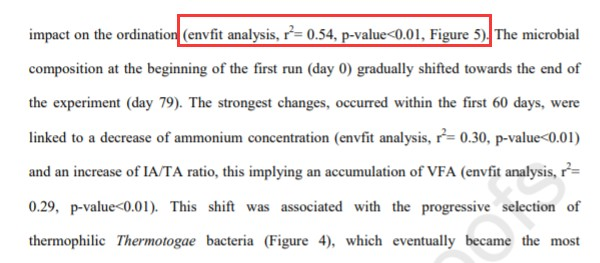### envfit()的线性拟合：非约束排序（PCA、CA、PCoA、NMDS）

envfit()的线性拟合

vegan中，可通过envfit()函数实现在非约束轴中被动拟合解释变量的过程，它是一种线性的方法。由于envfit()只能与vegan包中的排序函数一起配合使用，下文对四种常见的非约束排序（PCACAPCoANMDS）都简介一下。

`#加载 vegan 包library(vegan) #读入物种多度数据phylum <- read.delim('phylum_table.txt', row.names = 1, sep = '\t', stringsAsFactors = FALSE, check.names = FALSE) #读取环境数据env <- read.delim('env_table.txt', row.names = 1, sep = '\t', stringsAsFactors = FALSE, check.names = FALSE)`

PCA排序

`##tb-PCA#物种数据 Hellinger 预转化phylum_hel <- decostand(phylum, method = 'hellinger') #PCA 排序tbpca <- rda(phylum_hel, scale = FALSE) #查看 PCA 结果等，I 型标尺为例summary(tbpca, scaling = 1)plot(tbpca, choices = c(1, 2), scaling = 1,  display = c('wa'))`

`#环境变量与非约束轴的多元回归，999 次置换检验，详情 ?envfit()tbpca_ef <- envfit(tbpca~., data = env, perm = 999, choices = c(1,2), display = 'sites')tbpca_ef`

tbpca_ef”的结果如下所示。与RDA相似，环境变量投影到排序图中以向量的形式作为展示，下图中PC1PC2即分别为各环境变量向量的顶点在PCA第一轴和第二轴中的坐标；r2为多元回归的回归决定系数R2Pr(>r)即显著性p值。

`#p 值校正，bonferroni 方法tbpca_ef_adj <- tbpca_eftbpca_ef_adj\$vectors\$pvals <- p.adjust(tbpca_ef_adj\$vectors\$pvals, method = 'bonferroni')tbpca_ef_adj`

p值校正后，对于上述示例，最终仅保留了4个显著的环境变量。而且我们发现，这4个环境变量与PCA非约束轴样方得分之间存在较为可观的线性关系（根据R2判断）。

`#提取样方得分（坐标），I 型标尺为例，前两轴tbpca_site.scaling1 <- scores(tbpca, choices = 1:2, scaling = 1, display = 'sites')#或者tbpca_site.scaling1 <- summary(tbpca, scaling = 1)\$sites[ ,1:2]#若输出在本地，以 csv 格式为例write.csv(data.frame(tbpca_site.scaling1), 'pca_site.scaling1.csv', quote = FALSE) #提取物种得分（坐标），I 型标尺为例，前两轴tbpca_species.scaling1 <- summary(tbpca, scaling = 1)\$species[ ,1:2]#若输出在本地，以 csv 格式为例write.csv(data.frame(tbpca_species.scaling1), 'pca_species.scaling1.csv', quote = FALSE) #提取特征根、各轴解释量（各轴特征根占总特征根的比值）eig <- tbpca\$CA\$eigeig_prop <- tbpca\$CA\$eig / sum(tbpca\$CA\$eig) #将环境变量的坐标、多元回归 r2、回归显著性（校正后的 p 值）输出在本地，以 csv 格式为例tbpca_env <- data.frame(cbind(tbpca_ef_adj\$vectors\$arrows, tbpca_ef_adj\$vectors\$r, tbpca_ef_adj\$vectors\$pvals))names(tbpca_env) <- c('PC1', 'PC2', 'r2', 'p.adj')write.csv(tbpca_env, 'pca_env.csv', quote = FALSE)`

`#计算环境变量与样方得分的相关性（只计算显著的 4 个环境变量）tbpca_env_cor <- cor(env[ ,c('DOC', 'AP', 'AK', 'NH4')], tbpca_site.scaling1, method = 'pearson') #或者由排序坐标和 r2 反向推算出环境变量与样方得分的相关性arrow_heads <- tbpca_ef_adj\$vectors\$arrows       #提取 env 向量（坐标）矩阵r2 <- tbpca_ef_adj\$vectors\$r #提取 env r2arrow_heads * sqrt(r2)   #即可得相关性，结果和上述“tbpca_env”是一致的`

`#可使用 ?plot.cca 了解作图命令的更多参数信息，注意不要使用 ?plot #简要作图展示 PCA 结果（仅展示样方排序，不展示物种排序）plot(tbpca, choices = c(1, 2), scaling = 1, type = 'n')points(tbpca, choices = c(1, 2), scaling = 1, display = 'sites', pch = 20, col = c(rep('red', 9), rep('orange', 9), rep('green3', 9))) #作图展示被动拟合环境变量后的 PCA 结果，只保留 p.adj < 0.05 的环境变量plot(tbpca_ef_adj, choices = c(1, 2), p.max = 0.05)`

`##ggplot2 作图示例library(ggplot2) #提取样方和环境变量排序坐标，前两轴，I 型标尺tbpca_site.scaling1 <- data.frame(summary(tbpca, scaling = 1)\$sites[ ,1:2])tbpca_env_sign <- tbpca_env[which(tbpca_env\$p.adj < 0.05),1:2] #PCA 前两轴贡献度（%）tbpca_eig <- round(100 * tbpca\$CA\$eig[1:2] / sum(tbpca\$CA\$eig), 2) #读取样本分组数据（附件“group.txt”，第一列为各样本名称，第二列为各样本所对应的分组）group <- read.delim('group.txt', sep = '\t', stringsAsFactors = FALSE, check.names = FALSE) #合并样本分组信息，构建 ggplot2 作图数据集tbpca_site.scaling1\$sample <- rownames(tbpca_site.scaling1)tbpca_site.scaling1 <- merge(tbpca_site.scaling1, group, by = 'sample') tbpca_env_sign\$sample <- NAtbpca_env_sign\$group <- rownames(tbpca_env_sign) #ggplot2 作图library(ggplot2) ggplot(tbpca_site.scaling1, aes(PC1, PC2)) +geom_point(aes(color = group)) +scale_color_manual(values = c('red', 'orange', 'green3')) +theme(panel.grid = element_blank(), panel.background = element_rect(color = 'black', fill = 'transparent'), legend.title = element_blank(), legend.key = element_rect(fill = 'transparent')) + labs(x = paste('PCA1: ', tbpca_eig, '%'), y = paste('PCA2: ', tbpca_eig, '%')) +geom_vline(xintercept = 0, color = 'gray', size = 0.5) + geom_hline(yintercept = 0, color = 'gray', size = 0.5) +geom_segment(data = tbpca_env_sign, aes(x = 0,y = 0, xend = PC1/5, yend = PC2/5), arrow = arrow(length = unit(0.2, 'cm')), size = 0.5, color = 'blue') +geom_text(data = tbpca_env_sign, aes(PC1/5 * 1.1, PC2/5 * 1.1, label = group), color = 'blue', size = 3)`

CA、PCoA、NMDS等中的方法

vegan中，CA使用cca()执行，详情参考前文CA

`ca <- cca(phylum)`

vegan中，PCoA使用cmdscale()执行，详情参考前文PCoA

`##PCoA 排序#计算相异矩阵，以 Bray-curtis 距离为例bray <- vegdist(phylum, method = 'bray') #PCoA 排序pcoa <- cmdscale(bray, k = (nrow(phylum) - 1), eig = TRUE) #被动添加环境变量的过程和上述 tb-PCA 类似，简要演示#环境变量与非约束轴的多元回归，999 次置换检验pcoa_ef <- envfit(pcoa~., data = env, perm = 999, choices = c(1,2), display = 'sites')#p 值校正pcoa_ef\$vectors\$pvals <- p.adjust(pcoa_ef\$vectors\$pvals, method = 'bonferroni')#PCoA 作图plot(scores(pcoa)[ ,1], scores(pcoa)[ ,2], pch = 20, col = c(rep('red', 9), rep('orange', 9), rep('green3', 9)))#将 p.adj < 0.05 的结果投影至 PCoA 排序图plot(pcoa_ef, choices = c(1, 2), p.max = 0.05)`

vegan中，NMDS使用metaMDS()执行，详情参考前文NMDS

`##NMDS 排序，以 Bray-curtis 距离为例（vegan 包中 metaMDS() 执行，排序轴设定为 2）nmds <- metaMDS(phylum, distance = 'bray', k = 2)#被动添加环境变量的过程和上述 tb-PCA 类似，简要演示#环境变量与非约束轴的多元回归，999 次置换检验nmds_ef <- envfit(nmds~., data = env, perm = 999, choices = c(1,2), display = 'sites')#p 值校正nmds_ef\$vectors\$pvals <- p.adjust(nmds_ef\$vectors\$pvals, method = 'bonferroni')#NMDS 作图，仅展示样方排序，不展示物种排序plot(nmds, choices = c(1, 2), type = 'n')points(nmds, choices = c(1, 2), pch = 20, col = c(rep('red', 9), rep('orange', 9), rep('green3', 9)))#将 p.adj < 0.05 的结果投影至 NMDSplot(nmds_ef, choices = c(1, 2), p.max = 0.05)`

ordisurf()的非线性拟合

`##将环境变量以非线性曲面的形式投影至排序空间中，可借此探索排序样本沿环境变量的梯度分布#以两组环境变量（DOC、AK）为例plot(tbpca, choices = c(1, 2), scaling = 1,  display = c('wa'), main = 'DOC + AK')gam1 <- ordisurf(tbpca, env[, 'DOC'], add = TRUE, col = 'red3')gam2 <- ordisurf(tbpca, env[, 'AK'], add = TRUE, col = 'green3')#可以再把上述 envfit() 的线性拟合结果添进去，只显示环境变量“DOC”和“AK”select <- which(rownames(tbpca_ef_adj\$vectors\$arrows) %in% c('DOC', 'AK'))tbpca_ef_adj_select <- tbpca_ef_adjtbpca_ef_adj_select\$vectors\$arrows <- tbpca_ef_adj_select\$vectors\$arrows[select, ]tbpca_ef_adj_select\$vectors\$r <- tbpca_ef_adj_select\$vectors\$r[select]tbpca_ef_adj_select\$vectors\$pvals <- tbpca_ef_adj_select\$vectors\$pvals[select]plot(tbpca_ef_adj_select, choices = c(1, 2), add = TRUE, col = c('red3', 'green3'))#作图结果默认绘制，以下简要查看回归详情gam1summary(gam1)`

vegan常见问题列表：http://vegan.r-forge.r-project.org/FAQ-vegan.html

vegan常见问题列表：http://vegan.r-forge.r-project.org/FAQ-vegan.html

`#使用 Hellinger 转化后的物种数据执行RDA（此时即tb-RDA），以 4 个环境变量数据为例rda_tb <- rda(phylum_hel~., env[ ,c('DOC', 'AP', 'AK', 'NH4')], scale = FALSE)#拟合环境变量（与样方得分）rda_ef_site <- envfit(rda_tb~., env[ ,c('DOC', 'AP', 'AK', 'NH4')], perm = 999, choices = c(1,2), display = 'sites')rda_ef_site\$vectors\$pvals <- p.adjust(rda_ef_site\$vectors\$pvals, method = 'bonferroni')#拟合环境变量（与物种得分）rda_ef_lc <- envfit(rda_tb~., env[ ,c('DOC', 'AP', 'AK', 'NH4')], perm = 999, choices = c(1,2), display = 'lc')rda_ef_lc\$vectors\$pvals <- p.adjust(rda_ef_lc\$vectors\$pvals, method = 'bonferroni')#作图观察plot(rda_tb, display = c('wa', 'cn'), col = 'blue')      #RDAplot(rda_ef_site, col = 'red')   #与 RDA 样方得分的拟合plot(rda_ef_lc, col = 'green3') #与 RDA 物种得分的拟合`https://www.sciencedirect.com/science/article/abs/pii/S0960852419316724

• 发表于 2020-11-22 20:03
• 阅读 ( 7443 )
• 分类：宏基因组

## 作家榜 »

1.omicsgene 613 文章
2.安生水 290 文章
3.Daitoue 167 文章
4.生物女学霸 120 文章
5.红橙子 78 文章
6.CORNERSTONE 72 文章
7.生信老顽童 54 文章
8.landy 37 文章